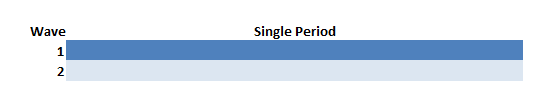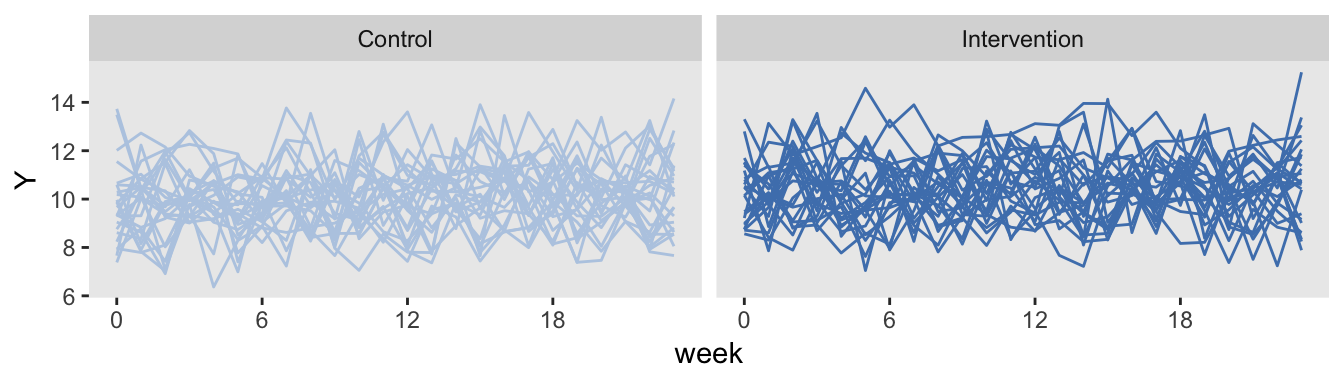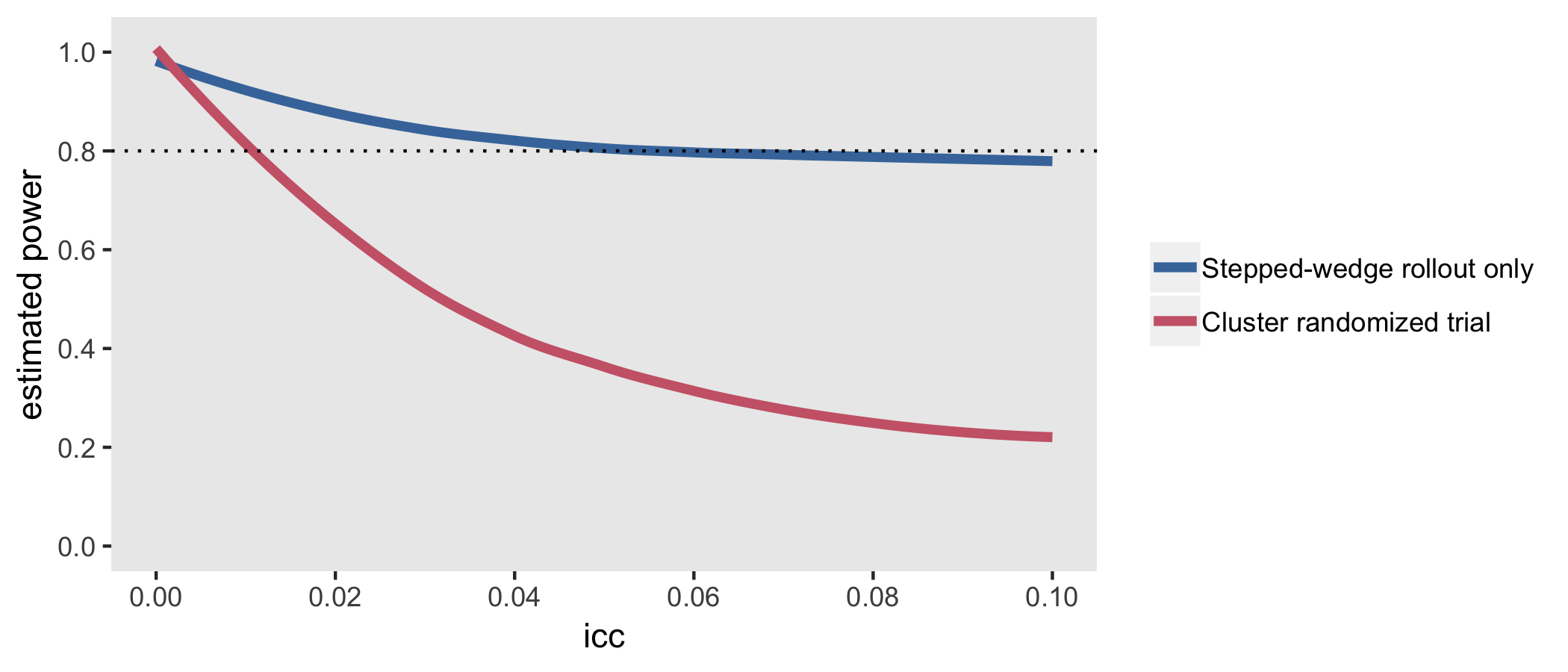# The power of stepped-wedge designs

Just before heading out on vacation last month, I put up a post that purported to compare stepped-wedge study designs with more traditional cluster randomized trials. Either because I rushed or was just lazy, I didn’t exactly do what I set out to do. I did confirm that a multi-site randomized clinical trial can be more efficient than a cluster randomized trial when there is variability across clusters. (I compared randomizing within a cluster with randomization by cluster.) But, this really had nothing to with stepped-wedge designs.

Here, I will try to rectify the shortcomings of that post by actually simulating data from a traditional stepped-wedge design and two variations on that theme with the aim of seeing which approach might be preferable. These variations were inspired by this extremely useful paper by Thompson et al. (If you stop reading here and go to the paper, I will consider mission accomplished.)

The key differences in the various designs are how many sites are exposed to the intervention and what the phase-in schedule looks like. In the examples that follow, I am assuming a study that lasts 24 weeks and with 50 total sites. Each site will include six patients per week. That means if we are collecting data for all sites over the entire study period, we will have $$24 \times 6 \times 50 = 7200$$ outcome measurements.

The most important assumption I am making, however, is that the investigators can introduce the intervention at a small number of sites during each time period (for example, because the intervention involves extensive training and there is a limited number of trainers.) In this case, I am assuming that at most 10 sites can start the intervention at any point in time, and we must wait at least 4 weeks until the next wave can be started. (We can proceed slower than 4 weeks, of course, which surprisingly may be the best option.)

I am going to walk through the data generation process for each of the variations and then present the results of a series of power analyses to compare and contrast each design.

### Stepped-wedge design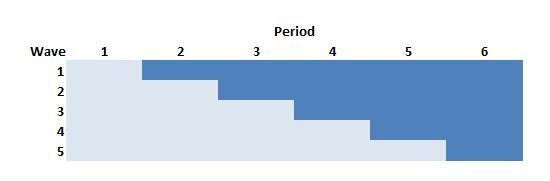In the stepped-wedge design, all clusters in a trial will receive the intervention at some point, but the start of the intervention will be staggered. The amount of time in each state (control or intervention) will differ for each site (or group of sites if there are waves of more than one site starting up at the same time).

In this design (and in the others as well) time is divided into discrete data collection/phase-in periods. In the schematic figure, the light blue sections are periods during which the sites are in a control state, and the darker blue are periods during which the sites are in the intervention state. Each period in this case is 4 weeks long.

Following the Thompson et al. paper, the periods can be characterized as pre-rollout (where no intervention occurs), rollout (where the intervention is introduced over time), and post-rollout (where the all clusters are under intervention). Here, the rollout period includes periods two through five.

First, we define the data, which will largely be the same across the designs: 6 individual patients per week, an intervention effect of 0.33, and a weekly time effect (which unfortunately is parameterized as “period”) of 0.02, and standard deviation within each cluster of 3.

library(simstudy)

defS <- defData(varname = "n", formula = 6,
dist = "nonrandom", id = "site")
defS <- defData(defS, varname = "siteInt", formula = 0,
variance = 1, dist = "normal")

formula = "(start <= period) * everTrt",
dist = "nonrandom")

formula = "10 + rx * 0.33 + period * 0.02 + siteInt",
variance = 9, dist = "normal")

Now, we actually generate the data, starting with the site level data, then the period data, and then the individual patient level data. Note that the intervention is phased in every 4 weeks so that by the end of the 24 weeks all 5 waves are operating under the intervention:

set.seed(111)

dS <- genData(50, defS)
dS[, start := rep((1:5)*4, each = 10)]
dS[, everTrt := 1]

dS[site %in% c(1, 2, 11, 12, 49, 50)] # review a subset
##    site n    siteInt start everTrt
## 1:    1 6  0.2352207     4       1
## 2:    2 6 -0.3307359     4       1
## 3:   11 6 -0.1736741     8       1
## 4:   12 6 -0.4065988     8       1
## 5:   49 6  2.4856616    20       1
## 6:   50 6  1.9599817    20       1
# weekly data
dP <- addPeriods(dtName = dS, nPeriods = 24, idvars = "site")

dP[site %in% c(3, 17) & period < 5] # review a subset
##     site period n     siteInt start everTrt timeID rx
##  1:    3      0 6 -0.31162382     4       1     49  0
##  2:    3      1 6 -0.31162382     4       1     50  0
##  3:    3      2 6 -0.31162382     4       1     51  0
##  4:    3      3 6 -0.31162382     4       1     52  0
##  5:    3      4 6 -0.31162382     4       1     53  1
##  6:   17      0 6 -0.08585101     8       1    385  0
##  7:   17      1 6 -0.08585101     8       1    386  0
##  8:   17      2 6 -0.08585101     8       1    387  0
##  9:   17      3 6 -0.08585101     8       1    388  0
## 10:   17      4 6 -0.08585101     8       1    389  0
# patient data
dI <- genCluster(dtClust = dP, cLevelVar = "timeID", numIndsVar = "n",
level1ID = "id")

dI
##       site period n   siteInt start everTrt timeID rx   id         Y
##    1:    1      0 6 0.2352207     4       1      1  0    1 10.810211
##    2:    1      0 6 0.2352207     4       1      1  0    2 14.892854
##    3:    1      0 6 0.2352207     4       1      1  0    3 12.977948
##    4:    1      0 6 0.2352207     4       1      1  0    4 11.311097
##    5:    1      0 6 0.2352207     4       1      1  0    5 10.760508
##   ---
## 7196:   50     23 6 1.9599817    20       1   1200  1 7196 11.317432
## 7197:   50     23 6 1.9599817    20       1   1200  1 7197  7.909369
## 7198:   50     23 6 1.9599817    20       1   1200  1 7198 13.048293
## 7199:   50     23 6 1.9599817    20       1   1200  1 7199 17.625904
## 7200:   50     23 6 1.9599817    20       1   1200  1 7200  7.147883

Here is a plot of the site level averages at each time point:

library(ggplot2)

dSum <- dI[, .(Y = mean(Y)), keyby = .(site, period, rx, everTrt, start)]

ggplot(data = dSum, aes(x = period, y = Y, group = interaction(site, rx))) +
geom_line(aes(color = factor(rx))) +
facet_grid(factor(start, labels = c(1 : 5)) ~ .) +
scale_x_continuous(breaks = seq(0, 23, by = 4), name = "week") +
scale_color_manual(values = c("#b8cce4", "#4e81ba")) +
theme(panel.grid = element_blank(),
legend.position = "none")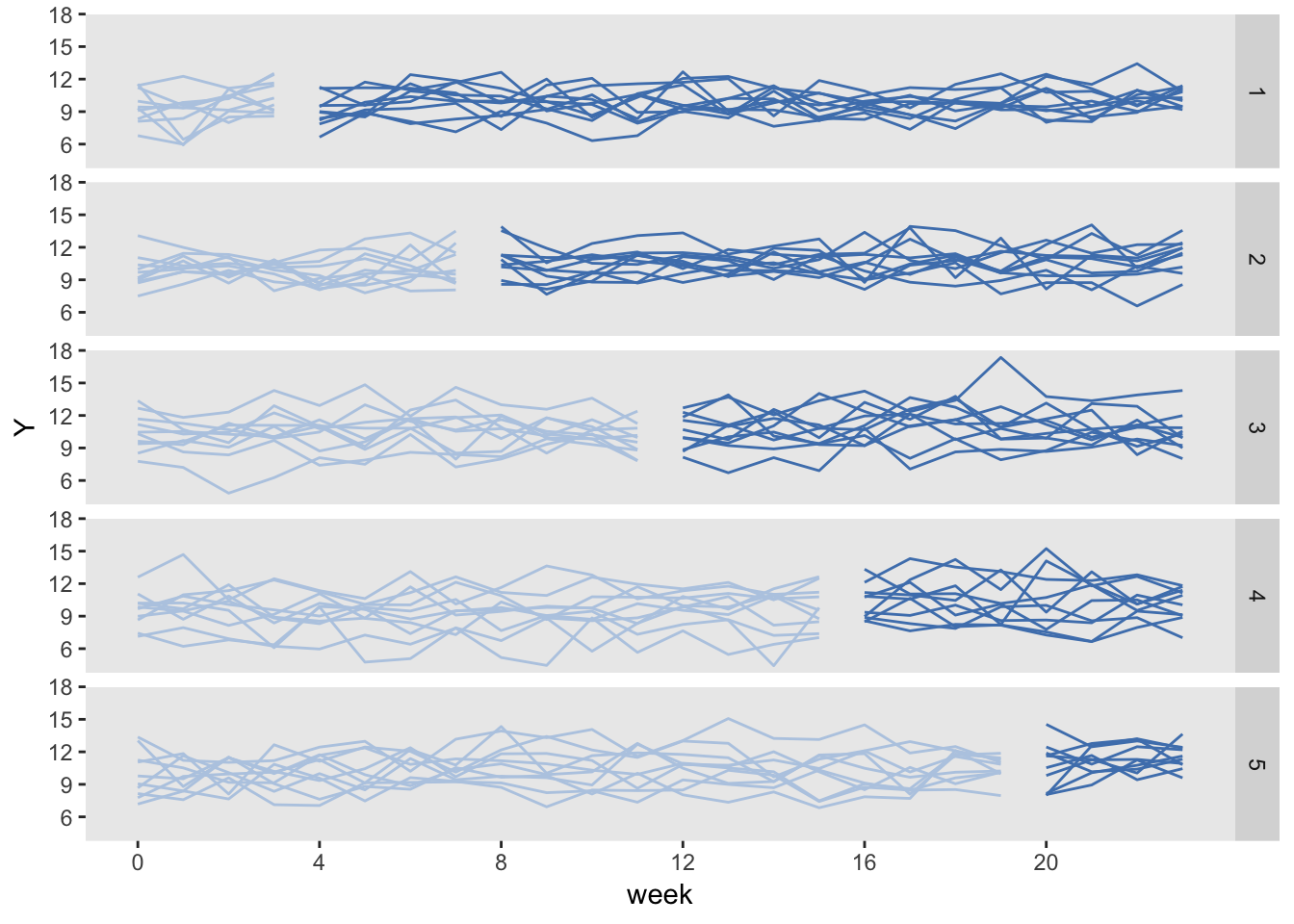Finally, we can fit a linear mixed effects model to estimate the treatment effect:

library(lme4)
library(broom)

tidy(lmer(Y ~ rx + period + (1|site), data = dI))
##                      term   estimate   std.error statistic    group
## 1             (Intercept) 9.78836231 0.184842722 52.955086    fixed
## 2                      rx 0.35246094 0.122453829  2.878317    fixed
## 3                  period 0.02110481 0.007845705  2.689983    fixed
## 4     sd_(Intercept).site 1.21303055          NA        NA     site
## 5 sd_Observation.Residual 2.99488532          NA        NA Residual

### Stepped-wedge using “rollout” stage only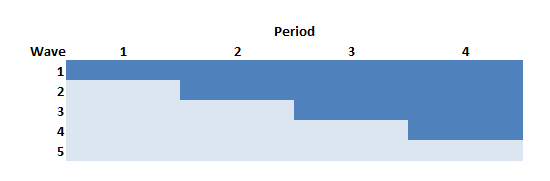The Thompson et al. paper argued that if we limit the study to the rollout period only (periods 2 through 5 in the example above) but increase the length of the periods (here, from 4 to 6 weeks), we can actually increase power. In this case, there will be one wave of 10 sites that never receives the intervention.

The data generation process is exactly the same as above, except the statement defining the length of periods (6 weeks instead of 4 weeks) and starting point (week 0 vs. week 4) is slightly changed:

dS[, start := rep((0:4)*6, each = 10)]

So the site level data set with starting points at 0, 6, 12, and 18 weeks for each of the four waves that ever receive treatment looks like this:

##    site n    siteInt start everTrt
## 1:    1 6  0.2352207     0       1
## 2:    2 6 -0.3307359     0       1
## 3:   11 6 -0.1736741     6       1
## 4:   12 6 -0.4065988     6       1
## 5:   49 6  2.4856616    24       1
## 6:   50 6  1.9599817    24       1

And the data generated under this scenario looks like: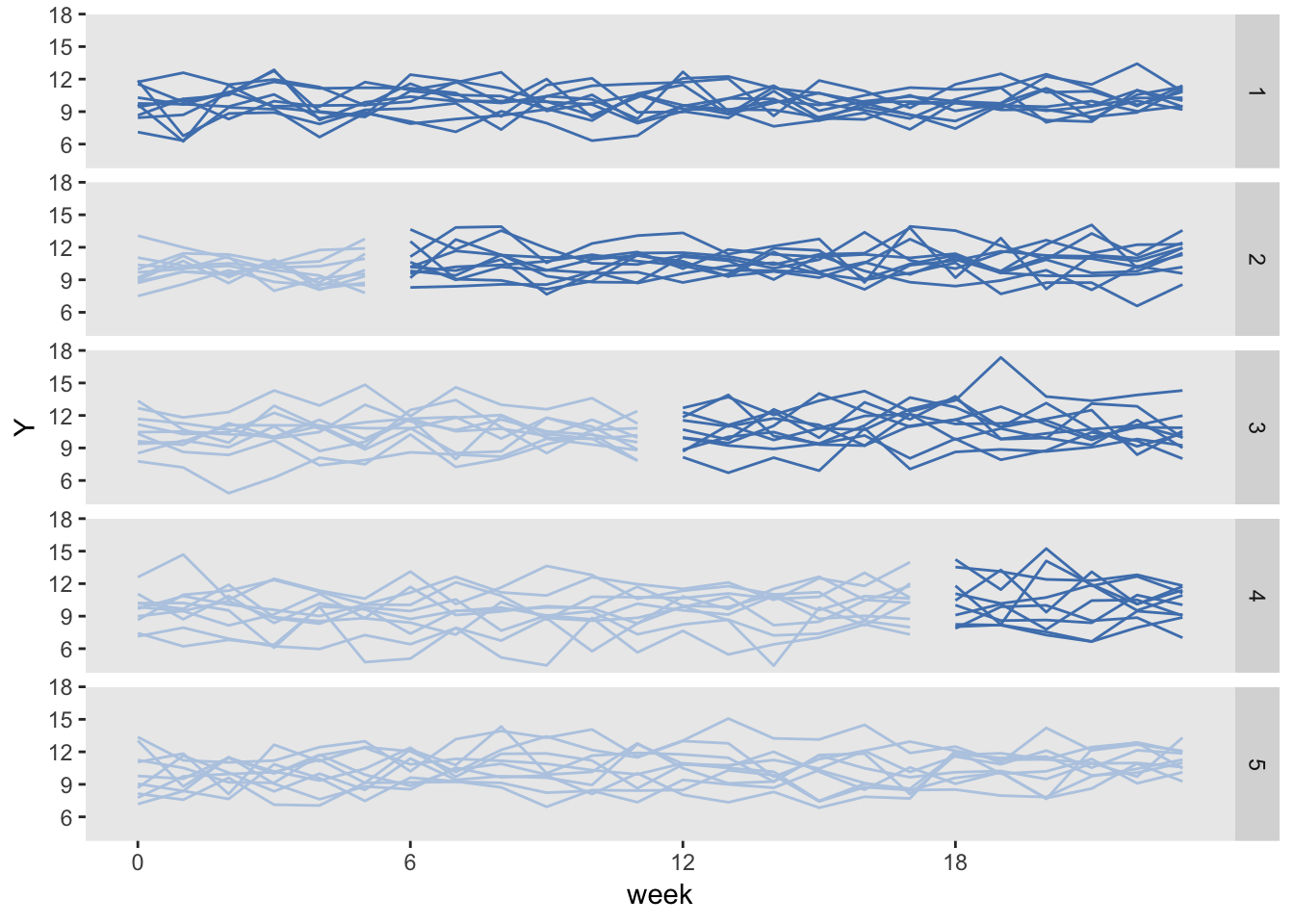Here is the model estimation:

tidy(lmer(Y ~ rx + period + (1|site), data = dI))
##                      term   estimate   std.error statistic    group
## 1             (Intercept) 9.79022407 0.185294936 52.835897    fixed
## 2                      rx 0.30707559 0.122414620  2.508488    fixed
## 3                  period 0.02291619 0.006378367  3.592800    fixed
## 4     sd_(Intercept).site 1.21153700          NA        NA     site
## 5 sd_Observation.Residual 2.99490926          NA        NA Residual

### Staggered cluster randomized trial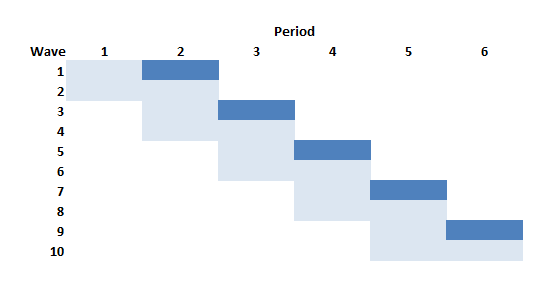If we wanted to conduct a cluster randomized trial but were able to phase in the intervention over time as we have been assuming, this design is the closest we could get. In this example with 50 sites and five phase-in periods, the intervention waves (in this example 1, 3, 5, 7, and 9) would each include five clusters. The respective control waves (2, 4, 6, 8, and 10) would also have five clusters each. And since we are assuming five waves, each wave will be in the study for eight: the first four weeks comprise “pre” measurement period, and the second four week period is the “post” measurement period.

The problem with this design relative to all the others discussed here is that the amount of data collected for each site is considerably reduced. As a result, this design is going to be much less efficient (hence less powerful) than the others. So much so, that I do not even generate data for this design (though I did actually confirm using simulations not shown here.)

### Staggered cluster randomized trial with continued measurement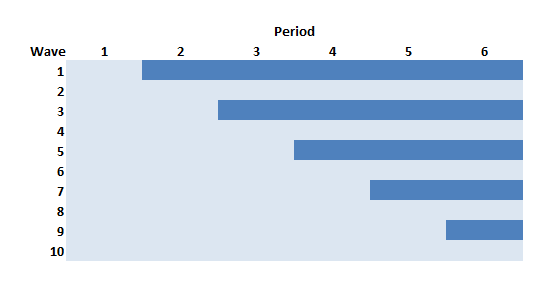This is the staggered CRT just described, but we collect data for all 24 weeks for all of the sites. In this case, we are not at disadvantage with respect to the number of measurements, so it might be a competitive design. This version of staggered CRT could also be viewed as a traditional stepped-wedge design with controls.

The data generation is identical to the traditional stepped-wedge design we started with, except the only half of the sites are “ever treated”:

dS[, everTrt := rep(0:1)]

Here is the plot, with the control arm on the left, and the intervention arm on the right. The control arm is never introduced to the intervention.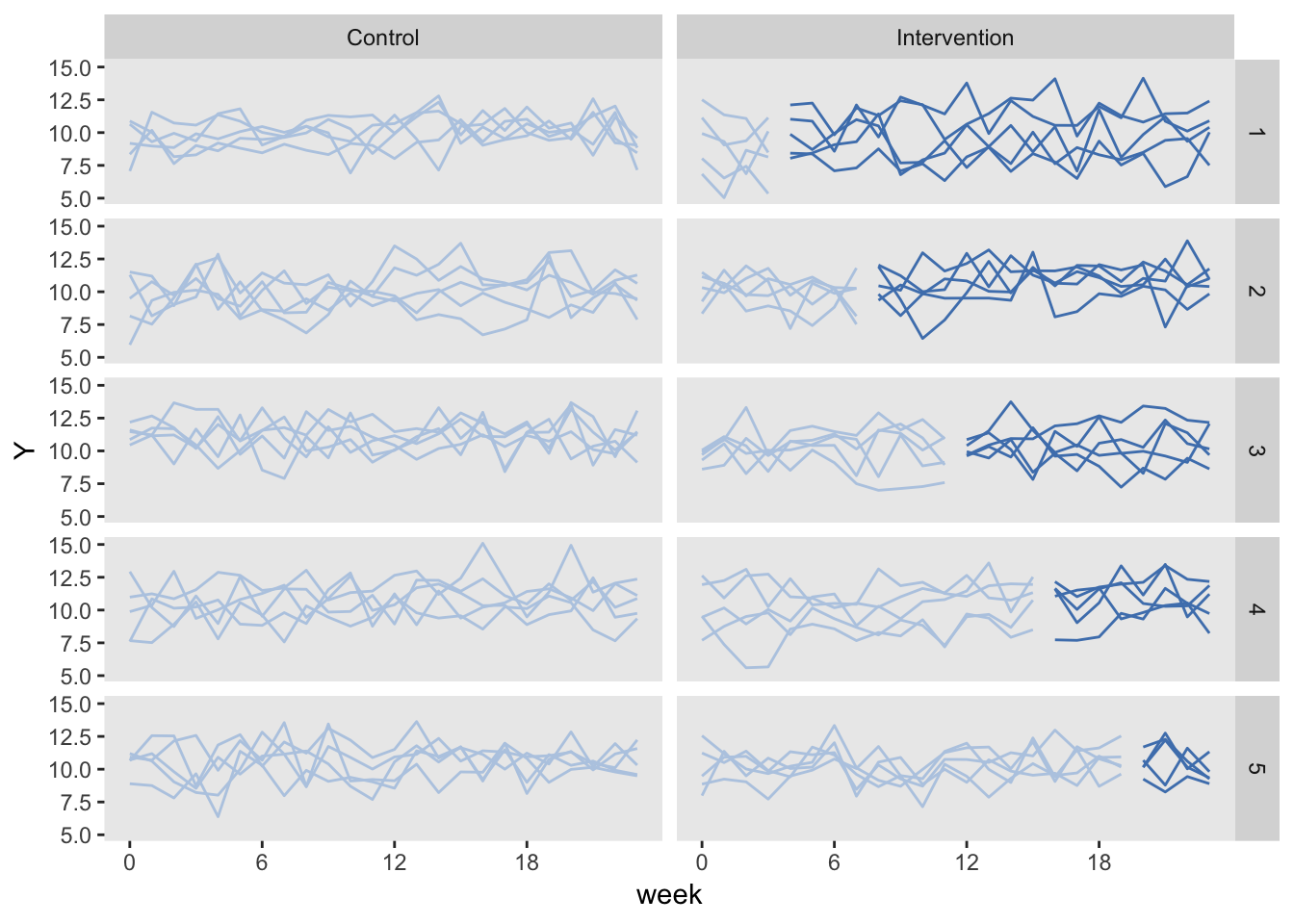### Conducting a power analysis using simulation

We are ultimately interested in assessing how much information each study design can provide. Power analyses under different conditions are one way to measure this.

Since one of my missions here is to illustrate as much R code as possible, here is how I do conduct the power analysis of the traditional stepped-wedge design:

powerStepWedge1 <- function(x) {

# generate data

dS <- genData(50, defS)
dS[, start := rep((1:5)*4, each = 10)]
dS[, everTrt := 1]

dP <- addPeriods(dtName = dS, nPeriods = 24, idvars = "site")

dI <- genCluster(dtClust = dP, cLevelVar = "timeID",
numIndsVar = "n", level1ID = "id")

# fit model

data.frame(summary(lmer(Y ~ rx + period + (1|site), data = dI))\$coef)
}

res <- vector("list", length = 5)
i <- 0

for (icc in seq(0, 0.04, .01)) {

i <- i + 1

# update data definition based on new ICC

between.var <- iccRE(ICC = icc, dist = "normal", varWithin = 9)
defS <- updateDef(defS, changevar = "siteInt", newvariance = between.var)

# generate 200 data sets and fit models
resSW1<- lapply(1:200, FUN = powerStepWedge1)

# estimate and store power
pSW1 <- mean( unlist(lapply(resSW1, [, 2, 3 )) >= 1.96)
res[[i]] <- data.table(icc, pSW1)

}

rbindlist(res)
##     icc  pSW1
## 1: 0.00 0.940
## 2: 0.01 0.855
## 3: 0.02 0.850
## 4: 0.03 0.830
## 5: 0.04 0.780

### Comparing power of three different designs

The next figure shows the estimated power for all three designs based on the same effect size and a range of ICC’s. The SW rollout only design consistently equals or outperforms the others. When the ICC is moderate to large (in this case > 0.06), the traditional SW design performs equally well. The design that comes closest to a staggered cluster randomized trial, the SW + controls performs well here on the lower range of ICCs, but is less compelling with more between site variation.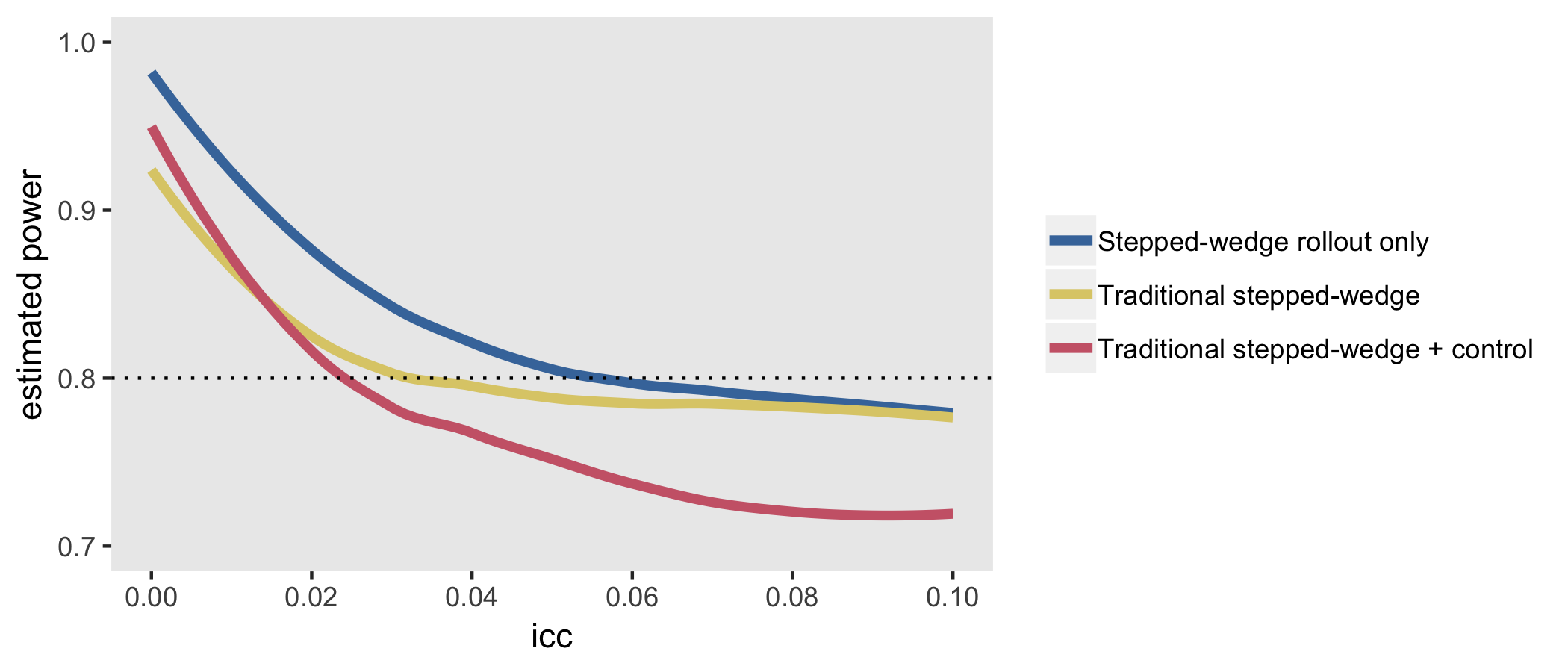Thompson et al. provide more nuance that can improve power under different conditions - mostly involving changing period lengths or adding control-only sites, or both - but these simulations suggest that some sort of stepped-wedge design (either limited to the rollout phase or not) will generally be advantageous, at least under the strict requirements that I established to frame the design.

All of this has been done in the context of a normally distributed outcome. At some point, I will certainly re-do this comparison with a binary outcome.